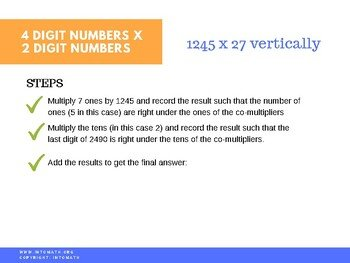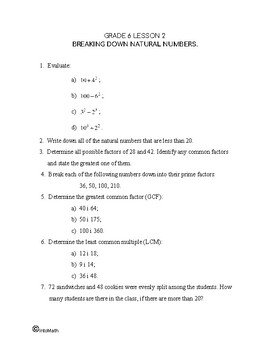CA\$2.00

In this lesson you will learn and practice how to multiply any number by a double-digit number vertically. For example, how to multiply 142 by 32.

You will also learn how to divide any number by a double-digit number using long division. For example, how to divide 345 by 21.

It is important to understand why place value of each digit within each number is important and why it is crucial to follow a certain form when performing operations in order to get the right answer.

In this lesson you will also discover division with a remainder.